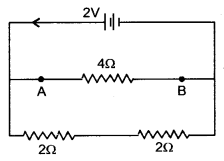# Electricity Class 10 Extra Questions with Answers Science Chapter 12

## Class 10 Science Chapter 12 Extra Questions and Answers Electricity

Extra Questions for Class 10 Science Chapter 12 Electricity with Answers Solutions

### Electricity Extra Questions Very Short Answer Type

Question 1.
The voltage-current (V-I) graph of a metallic conductor at two different temperatures T1 and T2 is shown in figure. At which temperature is the resistance higher?
At T2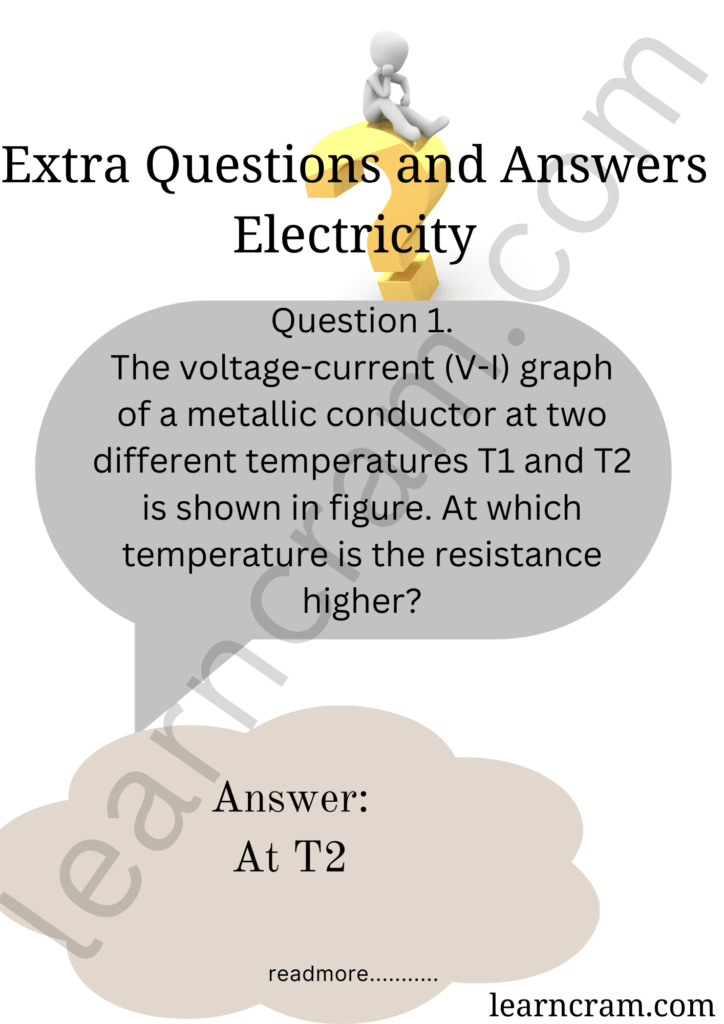Question 2.
Why is resistance less when resistors are joined in parallel?
We know that R ∝ $$\frac {1}{A}$$
In parallel combination of resistors, the effective area of cross-section of the conductor increases, so the resistance decreases.Question 3.
Why is resistance more in series combination?
We know R ∝ l. In series combination of resistors, the effective length of the conductor increases, so the resistance increases.

Question 4.
What are the special features of a heating wire?
It must have high specific resistance and high melting point.

Question 5.
What is the resistance of an ideal ammeter ?
Zero.

Question 6.
What is the resistance of an ideal voltmeter ?
Infinite.

Question 7.
There are 5 more electrons than the normal state of a conductor. What is the amount of charge on the conductor and its nature ? (Charge on electron, e = 1.6 × 10-19 C).
Given: n = 5, e = 1.6 × 10-19 C, Q = ?
Q = ne = 5 × 1.6 × 10-19 = 8.0 × 10-19C

Question 8.
Write the expression for the heat energy produced in a wire of resistance Rand carrying current I.
Heat produced, H = I2 Rt, where ‘t stands for time, for which current I is passed through the conductor.

Question 9.
Name the physical quantity whose unit is J/C.
Electric potential.

Question 10.
What is the resistance of an air gap?
It is very large, almost infinite.

Question 11.
In series combination which remains constant-current or voltage?
Current.

Question 12.
Which substance is used for making resistance coil of electric heater and
Nichrome, due to its high resistivity.

Question 13.
Name two devices in which electricity is converted into heat.
Electric heater and electric iron.

Question 14.
Name the alloy which is used for making the filament of bulbs.
Tungsten

Question 15.
Name the instrument used for measuring potential difference between two points in an electric circuit.
Voltmeter is used for measuring potential difference.

Question 16.
Name the instrument used for measuring electric reistivity of some materials: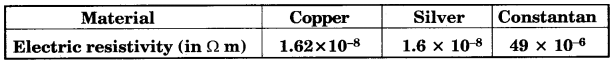Which one of these materials is the best conductor of electricity?
Out of these three, silver has the lowest resistivity. Hence, silver is the best conductor of electricity.

### Electricity Extra Questions Short Answer Type

Question 1.
Why does resistance of a metallic conductor increase with increase in temperature?
When a metallic conductor is heated, the atoms in the metal vibrate with greater amplitude and frequency. Due to increase in temperature, the thermal velocities of free electrons also increases. Therefore, the number of collisions between free electrons and atoms increases. This increases the opposition to the movement of electrons and hence the resistance of the conductor.

Question 2.
Why is it not advisable to handle high voltage electrical circuit with wet hands?
The resistance of dry-skin human body is about 50,000 Ω. When the skin is wet, the resistance gets lowered to about 10,000 Ω. If a person with wet hands touches the electrical circuit, high current will flow through the body causing risk of life.

Question 3.
Should the resistance of an ammeter be low or high ? Give reason.
It should be as close to zero as possible. Idéally it should be zero ohm. If it is non-zero and substantial it will after the true current.

Question 4.
Prove that the power of an electric bulb is invensly proportional to its resis tance.
Electric Power: It is defined as the amount of electric energy consumed in a circuit per unit time.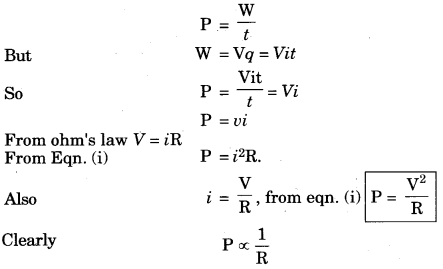Question 5.
Heat is generated continuously in an electric heater but the temperature of its element becomes constant after some time. Why?
When the temperature of the heater becomes greater than the temperature of the surrounding, some of the heat is lost to the surroundings in the form of thermal radiations.
After some time, rate at which heat is being produced becomes equal to the rate at which heat is lost. Hence, the temperature of the element becomes constant.

Question 6.
Two wires of equal length, one of copper and the other of manganin (an alloy) have the same thickness. Which one can be used for
(i) electrical transmission lines
(ii) electrical heating devices? Why?
(i) Copper wire can be used for electrical transmission lines because copper has very low resistivity and hence it is very good conductor of electricity.
(ii) Manganin can be used for electrical heating devices because the resistivity of manganin is about 25 times more than that of copper and hence it produces a lot of heat on passage of current through it.

Question 7.
What is electrical resistivity ? In a series electrical circuit comprising of a resistor having a metallic wire, the ammeter’ reads 5A. The reading of the ammeter decreases to half when the length of the wire is doubled. Why?
The resistivity of a material is defined as the resistance of a conductor made of that material of unit length and unit cross-sectional area.
Using, R = ρ $$\frac {l}{A}$$ Also, V = RI
R is doubled while V remains unchanged. Hence, current becomes half.

Question 8.
How does use of a fuse wire protect electrical appliances?
If a current larger than a specified value flows in a circuit, temperature of fuse wire increase its melting point. The fuse wire melts and the circuit breaks.

Question 9.
Why does an electric bulb become dim when an electric heater in parallel circuit is switched on? Why does dimness decrease after some time?
The resistance of a heater coil is less than that of electric bulb filament. When heater is switched on in parallel, more current start flowing through the heater coil and current through the bulb filament decreases making it dim.
After some time, when heater coil becomes hot its resistance increases. As a result, current through the heater coil decreases and the current through the bulb filament increases and thus dimness of the bulb decreases.

Question 10.
A metallic wire of resistance R is cut into ten parts of equal length. Two pieces each are joined in series and then five such combinations are joined in parallel.
What will be the effective resistance of the combination ?
The resistance of a conductor is directly proportional to the length of the conductor. The resistance of the metallic wire, when it is cut into ten parts of equal length is
r = $$\frac {R}{10}$$
Two such pieces when joined in series, the equivalent resistance of these two parts
= r + r = 2r = $$\frac {2R}{10}$$
Series resistance of two parts = 2 × $$\frac {R}{10}$$ = $$\frac {R}{5}$$
such elements are connected in parallel. Therefore the total resistance R’ will be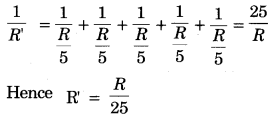Question 11.
Deduce an expression for equivalent resistance, if many resistons are con nected in parallel.
Parallel combination of Resistons: When two or more resistons are connected simultoneously between two point to each other then they are said to be connected in parallel combination. The potential difference across each resistor is same.
Total current is i = i1 + i2 + i3 ……… (i)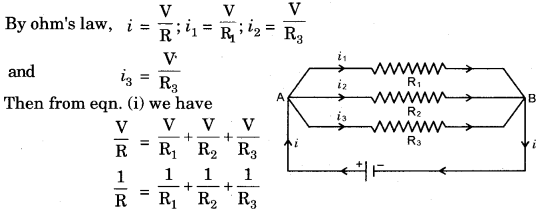Question 12.
What would be the reading of ammeter and voltmeter in the given circuit?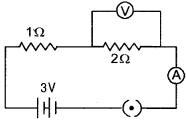R = R1 + R1 = 1 + 2 = 3 Ω
I = $$\frac {V}{R}$$ = $$\frac {3}{3}$$ = 1 A
Voltmeter rading = IR = 1 × 2 = 2V

Question 13.
(i) Resistivity of copper is lower than that of aluminium which in turn is lower than that of constantan.
(ii) Six wires labelled as A, B, C, D, E, F have been designed as per the followig parameters: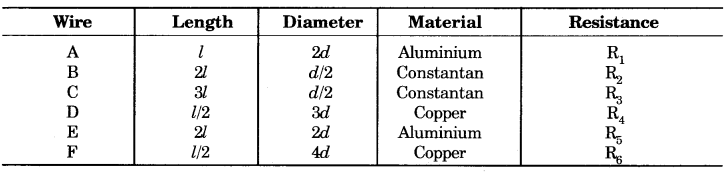Answer the following questions using the above data:
(a) Which of the wires has maximum resistance and why?
(b) Which of the wires has minimum resistance and why?
(c) Arrange R1, R3, and R5 in ascending order of their values. Justify your answer.
(a) Wire C has maximum resistance because it has maximum length, least thickness and highest resistivity.
(b) Wire F has the minimum resistance since it has least length, maximum tickness and least resistivity. (Using R = ρ $$\frac {l}{A}$$)
(c) R3 > R5 > R1 (Using relation R = ρ $$\frac {l}{A}$$ and comparing).

### Electricity Extra Questions Long Answer Type

Question 1.
(i) The potential difference between two points in an electric circuit is 1 volt. What does it mean? Name a device that helps to measure the potential difference across a conductor.
(ii) Why does the connecting cord of an electric heater not glow while the heating element does ?
(iii) Electrical resistivities of some substances at 20°C are given below:
Silver 1.60 × 10-8 Ω m
Copper 1.62 × 10-8 Ω m
Tungsten 5.2 × 10-8 Ω m
Iron 10.0 × 10-8 12 m
Mercury 94.0 × 10-8 Ω m
Nichrome 100 × 10-6 Ω m
Answer the following questions using above data:
(a) Among silver and copper, which one is a better conductor? Why?
(b) Which material would you advise to be used in electrical heating devices and why?
(i) The potential difference between two points is 1 volt means that if a charge of 1 coulomb is moved from one point to the other, 1 joule of work is required.
The potential difference across a conductor is measured by means of an instrument called voltmeter.

(ii) The electric power P is given by P = I2R
The resistance of the heating element very high. Large amount of heat generates in the heating element and it glows hot. The resistance of connecting cord is very low. Thus, negligible heat generates in the connecting cord and it does not glow.

(iii) (a) Silver is a better conductor due to its lower resistivity.
(b) Nichrome should be used in electrical heating devices due to very high resitivity.

Question 2.
Draw circuit diagrams to show how (i) flow of current and (ii) potential difference in a conductor is measured. Name apparatus used in it.
Measurement of Electric Current. Unit of current is ampere and instrument used for measuring current is called Ampere meter or simply Ammeter.It is a modified galvanometer having a low resistance connected in parallel to it. A moving coil galvanometer consists of a rectangular coil of wire of many turns, wound on a non-magnetic metallic light frame and is suspended between two strong permanent concave shaped magnetic poles. When current is allowed to pass through it a torque acts on the coil which deflects the coil. The deflection of the coil is directly propor-tional to the current flowing in the coil. The deflection of the coil is directly read on the scale with the help of a pointer attached with the coil. The scale is graduated in ampere or milliampere.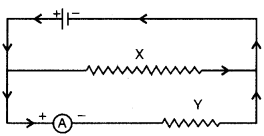To measure the current in a circuit, ammeter is connected in series in the circuit. It should be noted that positive terminal of the ammeter is connected with the positive terminal of the cell and negative terminal of the ammeter is connected with negative terminal of the cell.

If more than one conductors are connected in parallel in the circuit, then ammeter should be connected with that conductor in which current is to be measured. For example, for measuring current in the conductor Y, ammeter A is connected in series with the conductor Y (Fig.)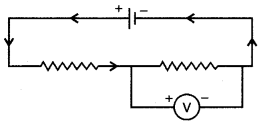Measurement of Potential Difference. Unit of potential difference is volt and instrument used for measuring potential difference is called voltmeter. It is a modified galvanometer with high resistance connected in series with it. Its circular scale is graduated in Volts.

Voltmeter is connected in parallel to the conductor across which potential differenece is to be measured.
In the fig., different conductors P, Q and Rare placed in the circuit. It shows the arrangement of voltmeter V to measure the potential difference, across conductor R and arrangement of ammeter A to measure current in the conductor P.While connecting voltmeter in the circuit, positive terminal to the voltmeter should be connected to the positive terminal of the cell and negative with the negative of the cell.

Question 3.
Explain electromotive force of a cell and write necessary formula for it. What is the function of electric cell in an electric circuit?
An electric cell is a device in which a constant difference in potential is maintained between the two conductors by a chemical reaction.
Electromotive force. Cell converts chemical energy to electrical energy which makes charges to move. Work done by a cell in moving unit charge around the circuit is called electromotive force of the cell.
If W work is done in taking a test charge go around the complete circuit of the cell, then e.m.f. of the cell is
E = $$\frac{\mathrm{W}}{q_{0}}$$
It is measured in volt. An electric cell is used as a source of electric current.

Question 4.
Give reasons of the following:
(a) Metals are good electric con-ductors.
(b) Glass is non-conductor.
(c) To measure the current in a circuit ammeter is connected in series in the circuit.(a) We know that all metallic substances have free electrons, which keep moving within the substance randomly in all possible directions. These free electrons are distributed uniformly all over the body and move randomly and hence, there is no net transfer to charge. But these free electrons can move through the metal, or can be transferred from one part to other under the action of Electron an electric force. This movement of electrons from one part to the other constitute an electric current.

(b) In glass charges are not free to move i.e., electric current cannot flow. So glass is non-conductor or insulator.
(c) To measure the current in a circuit, ammeter is connected in series so the complete current of the circuit will pass through ammeter.

Question 5.
Explain electric potential difference and derive its unit.
Potential Difference. If we have to lift a mass, we have to do some work for it. In the same way, to move a charge from one point to other some work has to be done. This work is measurement of the difference in electric potential energy of the two points.

“The work done in moving a unit positive charge from one point to the other against the electrical force measures potential difference between two points.
If Q coulomb charge is shifted from one point to other and W joule work is done, then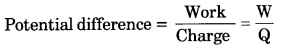The potential (or electrostatic potential) at any point is defined as the work done in bringing a unit positive charge from infinity to that point. Potential is generally denoted by the letter ‘V’ and its S.I. unit is ‘Volt’.

When 1 Joule of work is done in bringing 1 Coulomb positive charge from infinity to a point, potential at that point is 1 volt. Potential is a scalar quantity.
S.I. unit of potential difference is also ‘Volt’.
If W = 1 Joule, and Q = 1 Coulomb, then
V = 1 Volt.
The potential difference between two points is said to be 1 Volt if 1 joule of work is done in moving 1 Coulomb of charge from one point to another.

Question 6.
Three resistors of 2Ω 3, 3 Ω and 4 Ω are connected in: (i) Series, (ii) Parallel. Find the equivalent resistance in each case.
Solution.
Given: R1 = 2Ω, R2 = 3Ω and R3.= 4 Ω.
In series, the equivalent resistance is
Rg = R1 + R2 + R3 = 2 + 3 + 4 = 9 Ω
In parallel, if the equivalent resistance is Rp, then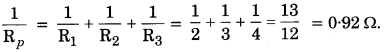Question 7.
Write Ohm’s law and describe the necessary experiment for its verification.
Ohm’s law. The physical conditions of a conductor remaining the same, the current flowing through the conductor is directly proportional to the potential difference between the ends of the con-ductor.
V = iR.
Verification of Ohm’s Law. Ohm’s law can be experimentally verified with the help of the electrical circuit shown in the diagram.AB represents a resistor or a piece of wire of resistance R. The following connections are made: .
(i) A voltmeter V is connected across (in parallel) the resistance R. It measures the potential difference across the resistance.

(ii) An ammeter or milli-ammeter (mA) is connected in series with the resistance. Therefore, the current pass-ing through the ammeter also passes through the resistance R

(iii) A rheostat is connected in series to change the resistance of the circuit so as to change the current in the circuit. To start the experiment, the key K is inserted so that a current begins to flow. This current is measured by the amme-ter. Initially, the rheostat is adjusted so that a small current flows. The reading of the ammeter gives the current flowing through the resistance and the voltmeter reading gives the potential difference across the resistance.

By adjusting the rheostat, the current is increased gradually and for each current value, the corresponding voltme-ter reading is noted. The ratio of potential difference to the current (V/i) is found for each reading and is found to be a constant. This also implies that the current is directly proportional to the potential difference. This verifies Ohm’s law experimentally.

Question 8.
Write the Precautions in the Use of Electricity.
Precautions in the Use of Electricity. The following precautions must be taken in the use of electricity:
(1) The circuit must be immediately switched off in case of fire or any other accident. This will prevent the fire from spreading.

(2) The person who happens to touch the live electric wire should be provided with an insulated support of wood, plastic or rubber. We should never try to pull away the person who is in contact with the live wire, otherwise we will also get a shock.

(3) All connections at switches, plugs and sockets and junctions must be tight. All wiring. should be of good quality, proper thickness, and standard insulation. Defective switches should be replaced immediately. All joints should be covered properly with appropriate insulating tape.

(4) The fuse should be of proper rating and material. Often connecting wires are used as fuses. This is a dangerous practice. Such wire has too high rating and will not protect you against a short circuit or overload.

(5) Whenever repairs are needed or any part of the circuit needs direct handling of any kind, rubber gloves and shoes must be used. The testers, screw drivers, pliers and other tools used for repairing, should have proper insulation.

### Electricity Extra Questions Numerical Type

Question 1.
A current of 0.5A is drawn by a filament of an electric bulb for 10 minutes. Find the amount of electric charge that flows through any point of the circuit.
It is given, I = 0.5A; t = 10 min = 600 s.
We know that, Q = It
= 0.5 A × 600 s = 300 C

Question 2.
A current of 5 A is flowing through a resistor of 15 Ω. Calculate the potential difference between the ends of the resistor.
Given, Resistance (R) = 15 ohms,
Current (I) = 5 amperes,
Potential difference (V) according to Ohm’s law
V = IR
⇒ V = 5 × 15 = 75 volt
∴ V = 75 volt

Question 3.
A piece of wire of resistance 20 Ω is drawn out so that its length is increased to twice its original length. Calculate the resistance of the wire in the new situation.
Suppose the length of R1 = 20 Ω resistance wire isl, its area of cross-section is A and its resistivity is ρ. Then,
R1 = $$\frac{\rho l}{A}$$ = 20 Ω
When length becomes twice (2l), then its area of cross-section will become half $$\left(\frac{A}{2}\right)$$. The new resistanceQuestion 4.
The resistance of a resistor is 4 Ω
when its length is I and cross sectional area A. Find the value of resistance of another resistor of some material of length 1/2 and cross sectional area 2A.Question 5.
A 4 Ω resistance wire is doubled on it. Calculate the new resistance of the wire.
We are given, R = 4 Ω
When a wire is doubled on it, its length would become half and area of cross-section would be doubled. That is, a wire of length $$\frac {l}{2}$$ becomes and area of cross-section 2A.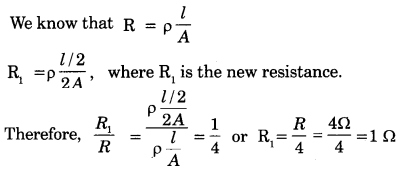The new resistance of the wire is 1 Ω.

Question 6.
A current of 1 ampere flows in a series circuit having an electric lamp and a conductor of 5 Ω when connected to a 10 V battery. Calculate the resistance of the electric lamp.
Now if a resistance of 10 Ω is connected in parallel with this series combination, what change (if any) in current flowing through 5 Ω conductor and potential difference across the lamp will take place? Give reason.(i) Let the resistance of the lamp = R1
512 Resistance of conductor = R2 = 5 Ω
Total resistance in series, Rs = R1 + R2 = R1 + 5
Current I = 1A, Voltage, V = 10 V
Using Ohm’s Law, V = IR
10 = 1(R1 + 5)
⇒ R1 = 5Ω

(ii) Now, a resistance of 10 Ω is connected in parallel with the series combination. Therefore, the total resistance of the circuit is given by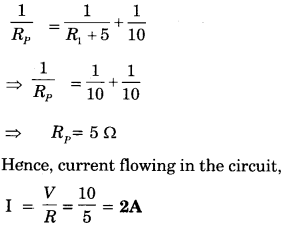Thus, 1 A current will flow through 10 Ω resistor and 1 A will flow through the lamp and conductor of 5 Ω resistance. Hence, there will be no change in current flowing through 5 Ω conductor. Also, there will be no change in potential difference across the lamp.

Question 7.
In the following diagram resistances are R1 = 15Ω, R2 = 10Ω, R3 = 30Ω, R4 = 5Ω, R5 = 20Ω and battery is 18 volt.Calculate the total resistance and the electrical current flowing in the circuit.
R1, R2, and R3 are connected in parallel, So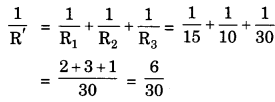∴ R’ = 5 Ω
R4 and R, are in Parallel. So,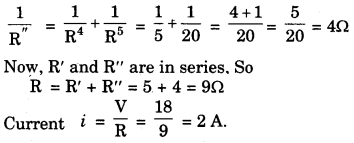Question 8.
In the given circuit, find the values of:
(i) Equivalent resistance
(ii) Total curent in circuit
(iii) Potential difference between A and B points.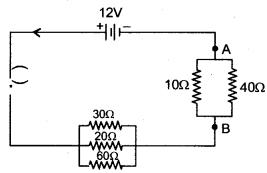(i) 30Ω, 20Ω and 60Ω are in parallel. So,(iii) Potential difference between points A and B is
V = i R” = 0.67 × 8 = 5.36 V.

Question 9.
Two resistances when connected in parallel give resultant value of 2 ohm, when connected in series the value becomes 9 ohm. Calculate the value of each resistance.
We know that two resistances are in parallel and hence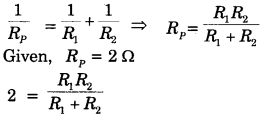2(R1 + R2) = R1R2 …..(1)
Now, same resistances are in series
Rs = R1 + R2
Given, Rs = 9 ohm
9 = R1 + R2 …..(2)
From (1) and (2), we get
R1 R2 = 18
Again using (2), we have
R2 = 9 – R1
∴ R1 (9 – R1) = 18Thus two resistance are 3 Ω and 6 Ω

Question 10.
A piece of wire of resistance R is cut into five equal parts. These parts are then connected in parallel. If the equivalent resistance of this combination is R’ then find the ratio $$\frac {R}{R}$$.
Resistance of a piece of length $$\frac {l}{5}$$ = $$\frac {R}{5}$$
The equivalent resistance of the 5 wires in parallel is R’. Then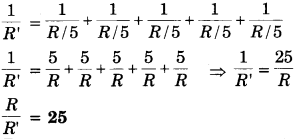Question 11.
Three 2 resistors A, B and C are connected as shown in figure. Each of them dissipates energy and can withstand a maximum power of 18 W without melting. Find the maximum current that can flow through the three reistors.
The current of resistor A is equally divided in resistors B and C; so maximum current can flow in resistor A.
Power = (current)2 × resistance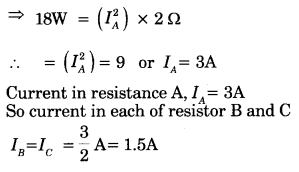Question 12.
0.50 A current is flowing through a electric bulb which is connected to 220V electric mains. Calculate the power.
Given:
i = 0.50A, V = 220 V, P = ?
P = Vi = 220 × 0.50 = 110 W

Question 13.
A geyser is rated 1500 W, 250 V. It is connected to 250 V mains. Calculate
(i) the current drawn
(ii) the energy consumed in 50 hours, and
(iii) the cost of energy consumed at Rs. 2.20 per kWh.
Given, V = 250 V, P = 1500 W, t=50 h = 50 × 60 × 60s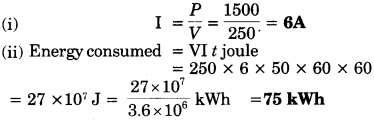(iii) ∵ 1 kWh costs = Rs. 2.20
∴ 75 kWh cost = 2.20 × 75 = Rs.165

Question 14.
Two resistors of 4 Ω and 6 Ω are connected in parallel. The combination is connected across a 6 volt battery of negligible resistance. Calculate
(i) the power supplied by the battery
(ii) the power dissipated in each resistor.
Here, R1 = 4Ω and R2 = 6 Ω
Both are in parallel. Hence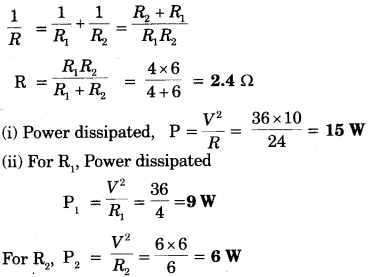Question 15.
Two lamps with rating constants 100 watt, 220 volt and 200 watt, 220 volts are connected in a parallel combination and with mains of electrical supply 220 volts. Calculate the current flowing through the mains.
Total power of the combination is
P = 100 + 200 = 300 W
∵ P = Vi
∴ i = $$\frac{P}{V}$$ = $$\frac{300}{220}$$ = 1.36 A.

Question 16.
Two conducting wires of the same material and of equal lengths and equal diameters are first connected in series and then in parallel in a circuit across the same potential difference. Find the ratio of heat produced in series and parallel combination.
Suppose the resistance of each one of the two wires is R.
The equivalent resistance of the series combination, Rs = R + R = 2R
The heat produced in time t,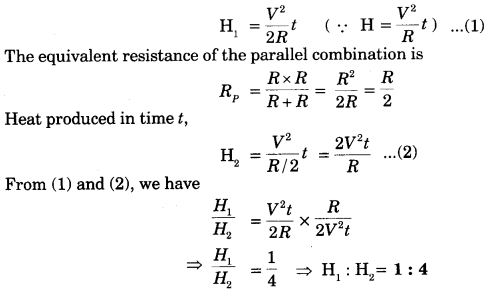Question 17.
Which has a higher resistance: a 50 W – 220 V lamp or a 25 W – 220V lamp? Calculate the ratio of their resistances.
Under a constant (fixed) applied voltage (V),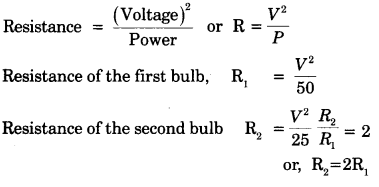R2 > R1, 25 W bulb has higher resistance. Resistance of 25 W lamp bulb is twice the resistance of 50 W lamp bulb.

Question 18.
An electric iron consumes energy at a rate of 840W when heating it at the maximum rate and 360W when the heating it at the minimum. The applied voltage is 220V. What is the value of current and the resistance in each case?
We know that the power input isQuestion 19.
The are two resistances of 3 ohm and 5 ohm, which of the resistances will be hatter if the two resistances joined (i) in resies and (ii) in parallel are connected to a cell?
When Both resistances are connected is series, the current will be same in these resistances. So, from the H = i2Rt, resistance of 5 Ω will be hotter.
When both resistances are connected in parallel, the voltage a cross the resistances is same. So, from the
H = $$\frac{\mathrm{V}^{2}}{\mathrm{R}}$$t , resistance of 3 Ω will be hotter.

Question 20.
A copper wire has diameter 0.5 mm and resistivity of 1.6 × 10-8 Ω-m. What will be the length of this wire to make its resistance 10 Ω? How much does the resistance change if the diameter is doubled?Question 21.
The values of current I flowing in a given resistor for the corresponding values of potential difference V across the resistor are given below:Plot a graph between V and I and calculate the resistance of that resistor.
V is kept on Y-axis and I on X-axis to plot a graph.Question 22.
When a 12 V battery is connected across an unknown resistor, there is a current of 2.5 mA in the circuit. Find the value of the resistance in the resistor.
We know,
R = $$\frac {V}{I}$$ (By Ohm’s Law)
V = 12 volts, I = 2.5 m A = 2.5 × 10-3 AQuestion 23.
If the resistivity of a copper wire is 1.6 × 10-8 Ω -m and diameter is 0.5 mm. What would be the length of wire if resistance is 10 Ω. If diameter of this is doubled, then what will be the change in resistance?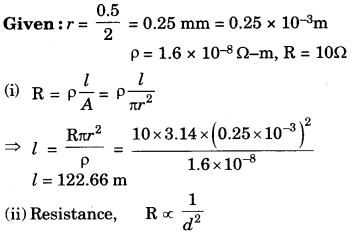If diameter is doubled, then resistance becomes one-fourth of its original value.

Question 24.
How many 176 Ω resistors (in parallel) are required to carry 5 A on a 220 V line?
Let the number of resistors = n.Number of resistors = 4

Question 25.
Show how would you connect three resistors, each of resistance 6 Ω, so that the combination has a resistance of (i) 9 Ω, (ii) 4 Ω.
We can connect resistance for (i) 9 Ω as below: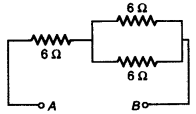This means that two resistors are connected in parallel, and one is connected in series to these two resistances to get 9 Ω resistance.
Again, for 4 Ω we will connect them as below: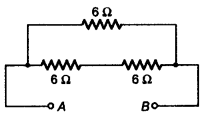Here, two resistances are connected in series, and this combination is connected to third resistance in parallel to get 4 Ω resistance.

Question 26.
Several electric bulbs designed to be used on a 220 V electric supply line, are rated 10 W. How many lamps can be connected in parallel with each other across the two wires of 220 V line if the maximum allowable current is 5A?
Resistance of each bulb = R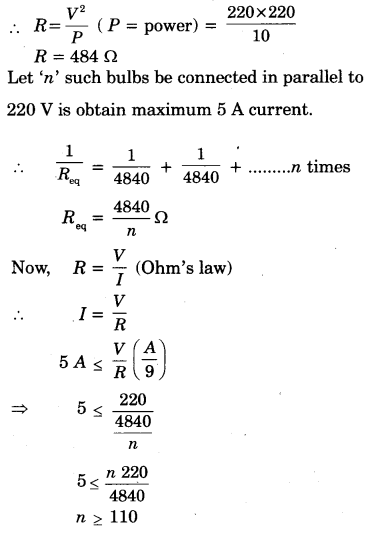∴ Number of electric bulbs = 110

Question 27.
A hot plate of electric oven connected to a 220 V line has two resistance coils A and B, each of 24 Ω resistance, which may be used separately, in series, or in parallel. What are the currents in the three cases ?
Case (i): When the plates are connected in series
R = 24 + 24 = 48 Ω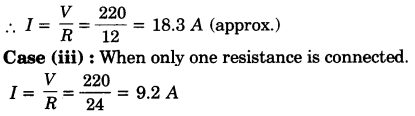Question 28.
Compare the power used in the 2Ω resistor in each of the following circuits:
(i) a 6 V battery in series with 1Ω and 2 Ω resistors, and
(ii) a 4 V battery in parallel with 12 Ω and 2 Ω resistors.Question 29.
Calculate the value of:
(i) Equivalent resistance
(ii) Total current in the circuit.
(iii) Potential difference between points A and B, in the following electrical cir cuit.